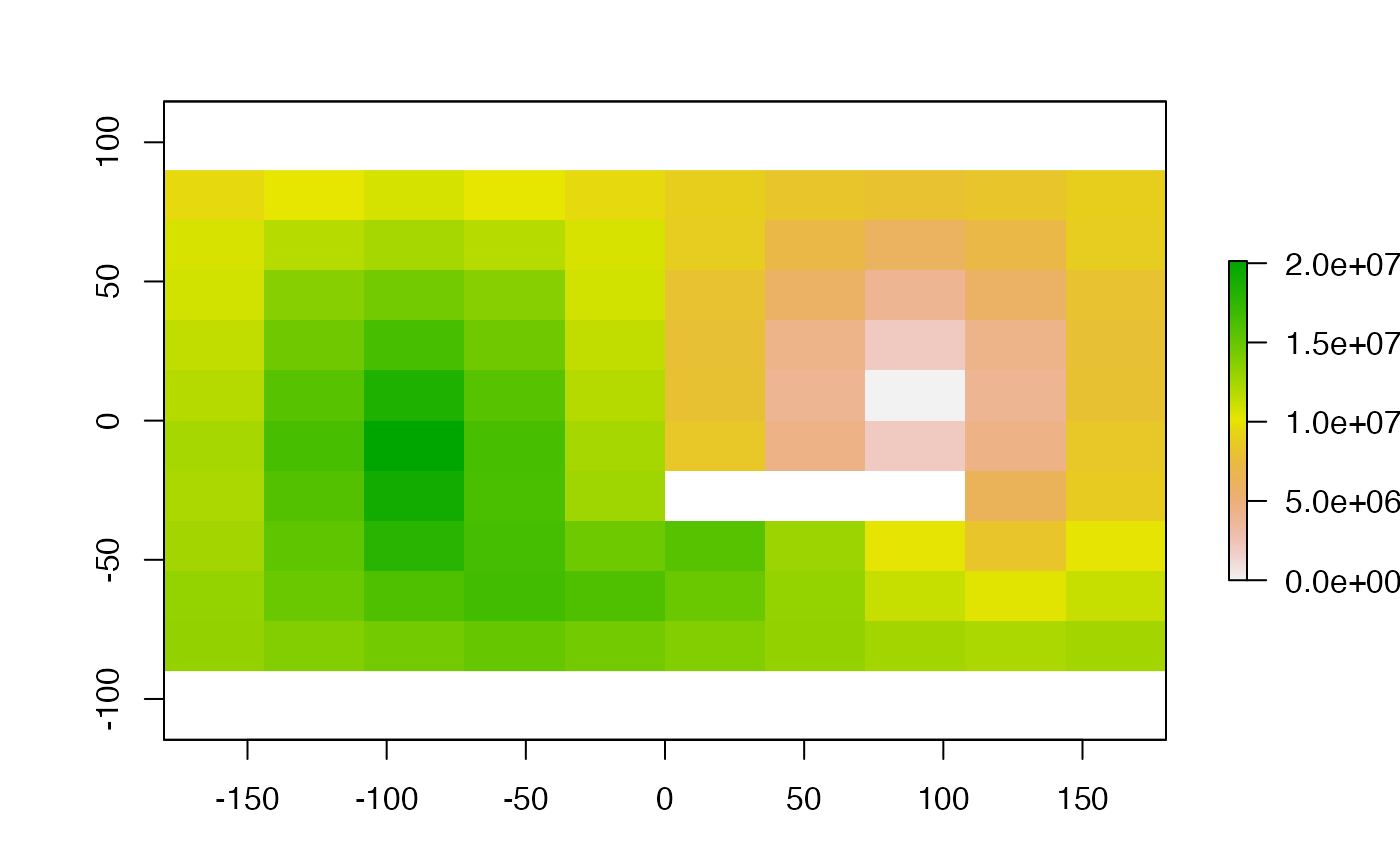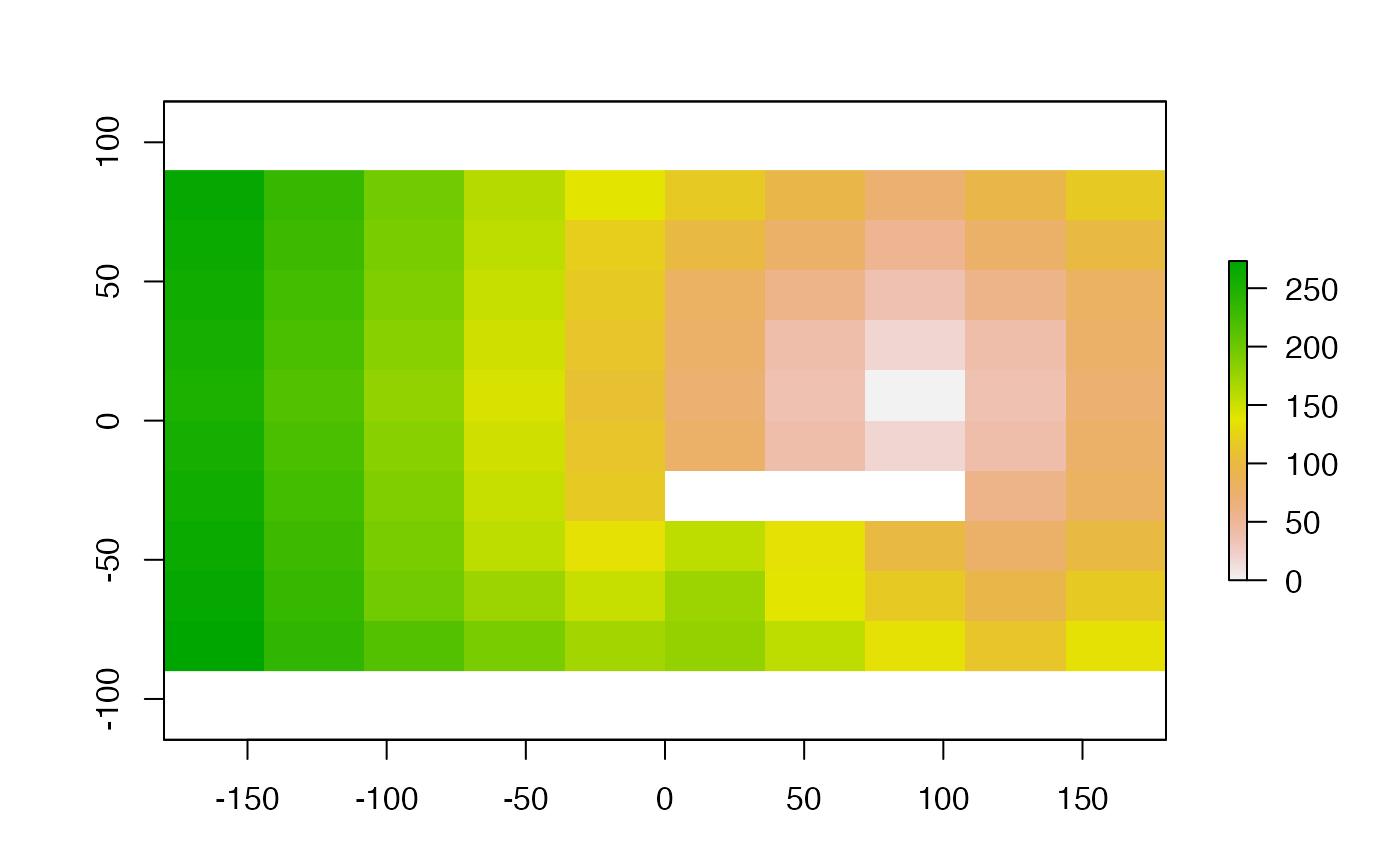The function calculates the distance to cells of a RasterLayer when the path has to go through the centers of neighboring raster cells (currently only implemented as a 'queen' case in which cells have 8 neighbors).

The distance is in meters if the coordinate reference system (CRS) of the RasterLayer is longitude/latitude (+proj=longlat) and in the units of the CRS (typically meters) in other cases.

Distances are computed by summing local distances between cells, which are connected with their neighbours in 8 directions.

# S4 method for RasterLayer
gridDistance(x, origin, omit=NULL, filename="", ...)

## Arguments

x

RasterLayer

origin

value(s) of the cells from which the distance is calculated

omit

value(s) of the cells which cannot be traversed (optional)

filename

character. output filename (optional)

...

additional arguments as for writeRaster

See distance for 'as the crow flies' distance. Additional distance measures and options (directions, cost-distance) are available in the 'gdistance' package.

## Details

If the RasterLayer to be processed is big, it will be processed in chunks. This may lead to errors in the case of complex objects spread over different chunks (meandering rivers, for instance). You can try to solve these issues by varying the chunk size, see function setOptions().

RasterLayer

## Author

Jacob van Etten and Robert J. Hijmans

## Examples


#world lon/lat raster
r <- raster(ncol=10,nrow=10, vals=1)
r <- 2
r[66:68] <- 3
d <- gridDistance(r,origin=2,omit=3)
plot(d)#UTM small area
crs(r) <- "+proj=utm +zone=15 +ellps=GRS80 +datum=NAD83 +units=m +no_defs"
d <- gridDistance(r,origin=2,omit=3)
plot(d)